# Simulation Study on Stress Intensity Factors of Surface Crack of Hollow Axle

Simulation Study on Stress Intensity Factors of Surface Crack of Hollow Axle

Haobo Lin Qiang Li Ran Ding

Engineering Research Center of Structure Reliability and Operation Measurement Technology of Rail Guided Vehicles, Ministry of Education, Beijing Jiaotong University, Beijing, China

Corresponding Author Email:
12116337@bjtu.edu.cn
Page:
179-183
|
DOI:
https://doi.org/10.18280/mmep.030405
N/A
|
Accepted:
N/A
|
Published:
31 December 2016
| Citation

OPEN ACCESS

Abstract:

Surface cracks may appear at the surface of hollow axles caused by accidental injury. Stress intensity factor is an important parameter to predict the crack growth. According to the European railway standards, using the finite element simulation software is to set the nominal stress of the axle surface to the maximum allowable stress and build finite element model of hollow axle surface crack. Calculate the stress intensity factors at different positions of the crack front. The distribution law of stress intensity factor of crack front expansion in different initial shape is obtained. The results show that with the crack growth, cracks with different initial shapes will extend to a certain range.

Keywords:

hollow axle, surface crack, stress intensity factor, finite element

1. Introduction

With the development of high speed railway at home and abroad, the application of hollow axle in railway vehicles will be more and more extensive. The axle is the key bearing part of locomotive and rolling stock bogie, its fatigue damage directly endangers the safety of transportation. Cutting shaft fracture [1, 2] is one of the main causes of railway vehicle overturning accident, it has a strong concealment and sudden and it brings huge losses and disasters to the national property and people’s security. In recent years, with the development of heavy load and high speed of the railway, axle will be affected by the increasingly severe impact inevitably. Especially cold cut shaft fracture phenomenon is more prominent.

After the cracks appear on the surface of the axle, the axle may break due to crack propagation when continue using. Predicting the fatigue crack propagation life is a problem that can prevent rupture of the axle and to determine whether the hollow axles with cracks continue to be used. And it is the basis of the research on interval time of nondestructive testing of axles. The fracture mechanics theory can be used to predict fatigue crack propagation life. Stress intensity factor is an important index in the analysis of fracture safety. It is the main parameter to judge whether a crack is produced under the load. On the calculation of stress intensity factor of three dimensional crack, there are engineering estimation method , finite element method , boundary integral method  and photo elastic method . Engineering estimation method is difficult to implement on some complex structure. The boundary integral method is lack of generality. Photo elastic experimental method is of high cost and long cycle. And the three-dimensional finite element method has been widely used in the calculation of stress intensity factor for its powerful simulation and numerical calculation.

2. Baisc Theory of Surface Crack Stress Intensity Factor Calculation Using Finite Element Method

2.1 Stress field and displacement field of the crack tip

According to the three-dimensional cracks in the component, it can be abstracted as the three-dimensional model represented by Figure 1. X direction is the crack front, Y direction is the normal direction of the crack surface, and Z direction is the direction of the tangent of a point on the crack. Consider a unit which is close to crack tip and in a polar coordinates. According to the analytical solution of elastic mechanics, the stress field can be expressed by the Eq (1).

## 1.png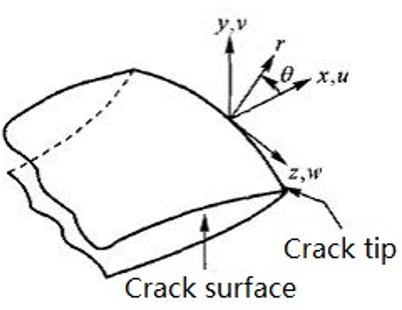Figure 1. 3D crack model

## eq1.png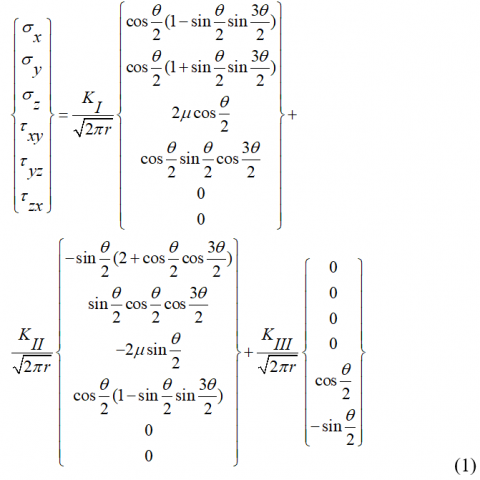According to the linear elastic fracture mechanics, the strain field in the crack tip region can be obtained by the formula of elastic mechanics, and then the displacement field in the crack tip can be expressed as:

$\left\{\begin{array}{l}u \\ v \\ w\end{array}\right\}=\frac{K_{I}}{4 G} \sqrt{\frac{r}{2 \pi}}\left\{\begin{array}{c}(2 k-1) \cos \frac{\theta}{2}-\cos \frac{3 \theta}{2} \\ (2 k+1) \sin \frac{\theta}{2}-\sin \frac{3 \theta}{2} \\ 0\end{array}\right\}+\frac{K_{I I}}{4 G}$

$\sqrt{\frac{r}{2 \pi}\left\{\begin{array}{c}(2 k+3) \sin \frac{\theta}{2}+\sin \frac{3 \theta}{2} \\ (3-2 k) \cos \frac{\theta}{2}-\cos \frac{3 \theta}{2} \\ 0\end{array}\right.}\}+\frac{K_{I I I}}{4 G} \sqrt{\frac{r}{2 \pi}}\left\{\begin{array}{c}0 \\ 0 \\ 8 \sin \frac{\theta}{2}\end{array}\right\}$          (2)

The r value is much smaller than the crack length. High-order terms have been omitted in Eq (1) and Eq (2). $\sigma_{x}, \sigma_{y}, \sigma_{z}, \tau_{x y}, \tau_{y z}$ and $\tau_{z x}$ are the stress components in the crack tip. u,v,x are arbitrary displacement components X, Y and Z of crack front in local rectangular coordinate system shown in Figure 1. $r, \theta$ are two coordinate components of local cylinder coordinate system shown in Figure 1.is the shear elastic modulus $(G=E / 2(1+\mu))$. k is a constant which has a certain relationship with material Poisson’s ratio $\mu$. For the plane strain problem, $k=3-4 \mu, \sigma_{z}=\mu\left(\sigma_{x}+\sigma_{y}\right)$. For the plane stress problem, $k=(3-\mu) /(1+\mu), \sigma_{z}=0 . \quad K_{I}$,  $K_{I I}$ and $K_{I I I}$  are stress intensity factors of type I, type II and type III.

2.2 Calculation of stress intensity factor

According to Eq (2), if one of the points on the crack surface is perpendicular to the crack plane, which displacement is known. Then the formula of the stress intensity factor for the symmetric crack is derived directly.

$\left\{\begin{array}{l}K_{I} \\ K_{I I} \\ K_{I I I}\end{array}\right\}=\lim _{r \rightarrow 0} \frac{2 G}{k+1} \sqrt{\frac{2 \pi}{r}}\left\{\begin{array}{c}v \\ u \\ \frac{k+1}{4} w\end{array}\right\}_{\theta=\pm\pi}$                                        (3)

In the bending stress state, the crack is usually extended by theⅠtype method. So the main research here is the surface crack ofⅠtype. A lot of studies have shown that the majority of the range along the crack front is in the plane strain state. This state can only exist in a very small place which is close to the free surface. From the plane strain state, the stress intensity factor and the displacement of crack surface of I type have the following relations.

$K_{I}=\frac{G v}{2(1-\mu)} \sqrt{\frac{2 \pi}{r}}=\frac{E v}{4\left(1-\mu^{2}\right)} \sqrt{\frac{2 \pi}{r}}$                               (4)

From the above stress field results, it can be concluded that the crack tip stress place has several characteristics.

1. At the crack tip, which is r=0, the stress approaches infinity.
2. Stress intensity factor K at the crack tip is limited.
3. The stress distribution near the crack tip is a function of r and θ.
3. Establishment of Crack Model

In the finite element analysis, in order to study the variation law of elliptical crack stress intensity factor on the surface of hollow shaft under load, the stress condition of hollow shaft should be determined. According to EN13103 , the maximum stress on axle surface is 180MPa. Using finite element calculation software ANSYS to simulate the maximum stress state on the axle surface.

To calculate the stress intensity factor at the crack tip, we must solve the modeling problem of the crack body [8-10]. In this paper, the crack front is used in the singular element with 20 1/4 nodes. The 1/4 node is used to replace the crack tip node in order to adapt to the stress singularity at the proper place. Figure 2 and Figure 3 are the crack front model and the mesh of the crack.

## 2.png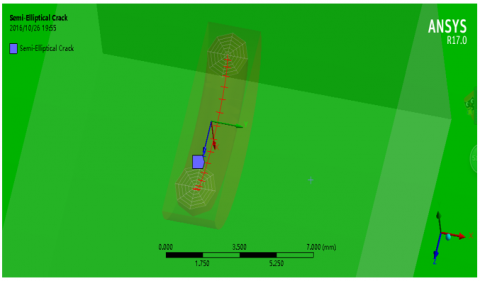Figure 2. Crack front model

## 3.png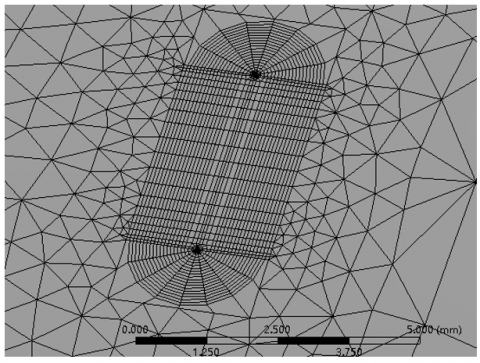Figure 3. Crack finite element

4. Finite Element Analysis of Semi Elliptical Crack Stress Intensity Factor on the Surface of Hollow Axle

In this paper, two dimensions of the axle model are established, respectively, the original size of the axle model and 3 to 1 smaller size of the axle model. Crack shape ratio is defined as the ratio of crack depth and elliptical crack long axis b. 5 different crack depths and 10 different shape ratios are analyzed. 5 different crack depths are 1mm, 2mm, 3mm, 4mm, 5mm, and 10 different shape ratios, respectively, 1/10, 1/9, 1/8, 1/7, 1/6, 1/5, 1/4, 1/3, 1/2, 1. The distribution of stress intensity factors of different crack shapes at different depths are calculated. The distribution of stress intensity factor of the crack front are shown in Figure 4, 5 and 6. Horizontal axis is normalized crack front distance, 0 and 1 respectively represent the two surface points of the crack.

It can be seen from the figure that the stress intensity factor of the crack at the end of the crack is larger than that middle of the crack in the case of the shape ratio of 1, regardless of the crack depth. This shows that the end of the semi-circular crack propagation speed is larger than the crack tip position, the semi-circular crack has the tendency to turn to elliptical crack. When the shape ratio is less than 1/2, the stress intensity factor of the crack tip is larger than that the end of crack. This shows that the straight crack also has the tendency to turn to elliptical crack. Also it can be seen that when the shape ratio is smaller than 1/5, the stress intensity factor is gradually close.

Stress intensity factor of 1mm and 2mm deep crack is fluctuating in the whole crack front. But it is more stable in the case of shape ratio 1 and 1/2. In 2mm, the results is close when shape ratio is between 1/2 and 1/4. But with the deepening of the crack, results of shape ratio 1/2 are different from other shape ratio.

Regardless of the crack depth and shape ratio size, the original size model simulation results are always larger than the 3 to 1 model simulation results. It proved that the small size model has better fracture mechanics properties. The variation law of the original size model is basically the same as that of the 3 to 1 dimensional model, which has good consistency.

## 4a.png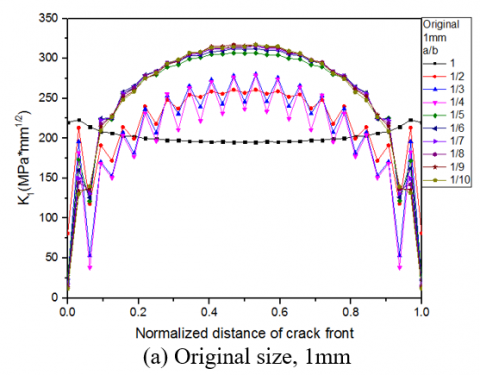## 4b.png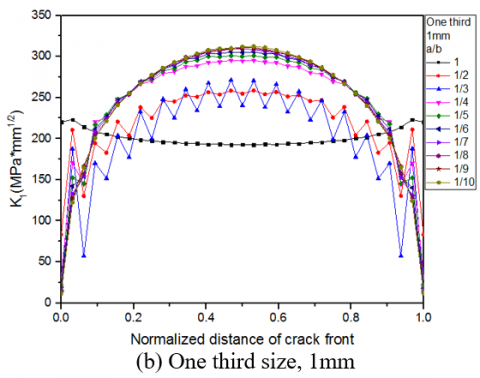## 4c.png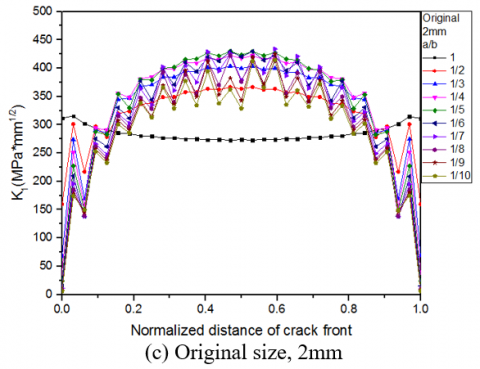## 4d.png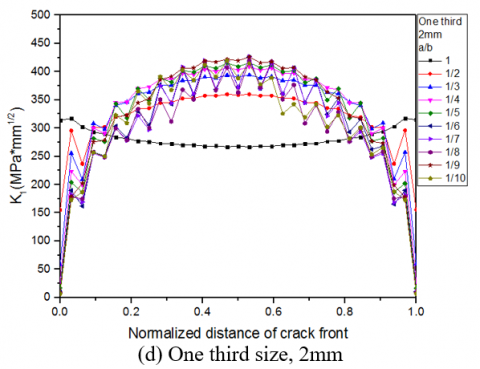## 4e.png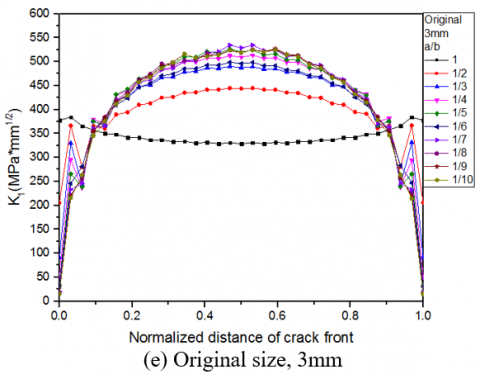## 4f.png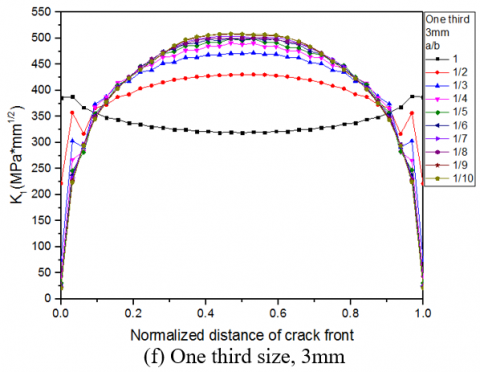## 4g.png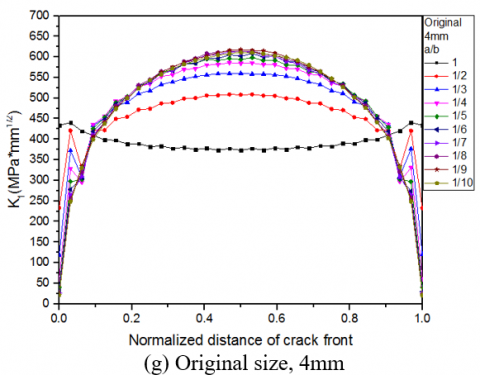## 4h.png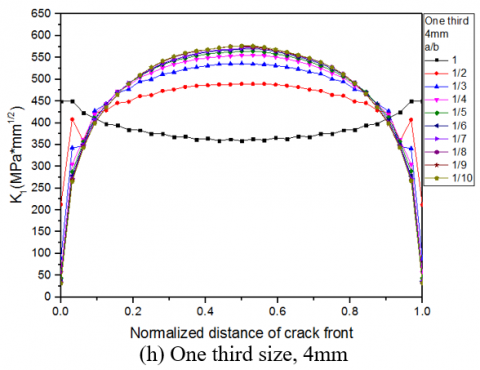## 4i.png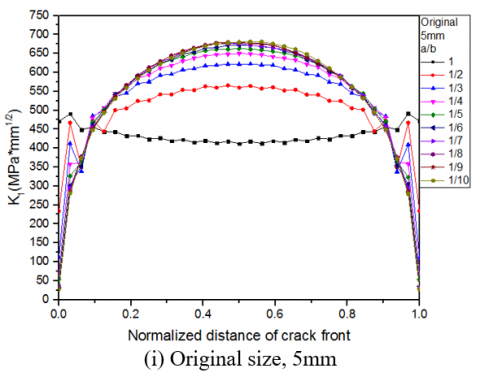## 4j.png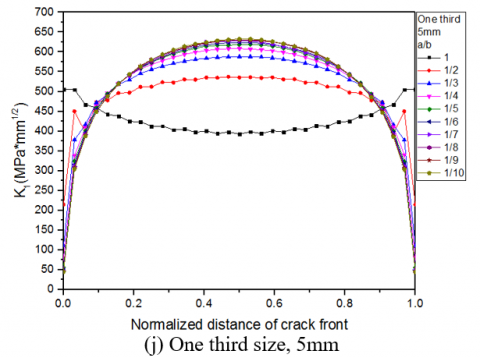Figure 4. Stress intensity factor distribution of crack front

## 5.png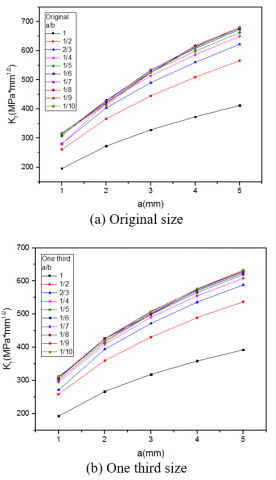Figure 5. Simulation solution of stress intensity factor of different shape crack with change of depth

## 6.png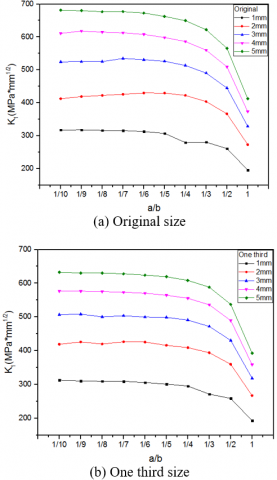Figure 6. Simulation solution of stress intensity factor of different depth crack with different shape ratio

5. Conclusion

In this paper, the stress state of the hollow axle is analyzed, and the finite element model of the crack on hollow axle surface is established. On the basis of model, the stress intensity factors of the crack front are calculated and analyzed. The distribution law of crack front stress intensity factor of different initial shape is obtained. The conclusions are as follows.

1. The results show that the finite element method is feasible and reliable to solve stress intensity factor of 3D surface crack.
2. The distribution of stress intensity factors at crack front under different shape ratio and different depth on the surface of the hollow axle is analyzed under the allowable stress. The distribution law of stress intensity factor of crack on hollow axle is obtained. It provides a good reference to study the fracture reliability of hollow axles.
Acknowledgment

This paper is supported by “The National Key Research and Development Program of China (2016YFB1200403)”.

Nomenclature
 $\sigma_{x}$ bending stress in X direction $\sigma_{y}$ bending stress in Y direction $\sigma_{z}$ bending stress in Z direction $\tau_{x y}$ shear stress in X direction $\tau_{y z}$ shear stress in Y direction $\tau_{z x}$ shear stress in Z direction G shear elastic modulus $\mu$ Poisson ratio $K_{I}$ type I stress intensity factor $K_{I I}$ type II stress intensity factor $K_{I I I }$ type III stress intensity factor
References

 Song Zilian and Shi Jianping, Analysis of Axle Fracture Failure, Beijing, China: China Railway Publishing House, 1995.

 Transport Bureau of the Ministry of Railways, Institute of metals and chemistry, Academy of Railway Sciences. Typical Damage Atlas of Railway Freight Cars.; Beijing, China: China Railway Publishing House, 2006.

 Wang, X. and Lambert S. B., “On the calculation of stress intensity factors for surface cracks in welded pipe-palte and tubular joints,” International Journal of Fatigue,vol 25, pp. 89-96, 2003.

 Manuel Da Fonte and ManueL de Freitas, “Stress intensity factors for semi—elliptical surface cracks in round bars under bending and torsion,” International Journal of Fatigue, vol. 21, pp. 457-463, 1999.

 Lie S. T., Li G. And Cen Z., “Effect of brace wall thickness and weld size on stress intensity factors for welded tubular T joints,” Journal of Constructional Steel Research, vol. 53, pp. 167—182, 2000．

 Li Xiang-Tin. Zhu Xiang-Hua and Xie Li-Xin, “Photoelastic determination of mixed-mode stress intensity factors in a cylindrical bar subjected to bending and torsion simultaneously,” Journal of Nanchang University (Engineering & Technology), vol. 27, no. 1, pp. 33-39, 2005.

 EN13103, Railway applications-Wheelsets and bogies -Non-powered axles -Design method, 2003.

 Lin Xiao-Bin and Roderick A Smith, “Calculation for the stress intensity factors based on three-dimensional limited element method,” China Mechanical Engineering, vol. 9, no 11, pp, 39-42, 1998.

 Chen Jia-Quan, Shen Wei-Liang and Xu Jia-Yuan, “Finite element calculation of stress intensive factor,” Equipment Manufacturing Technology, vol. 4, pp. 6-9, 2003.

 Chen Ai-Jun, Xu Cheng and Hu Xiao-qiu, “Several methods for calculating stress intensity factors of thick walled cylinder with cracks,” Journal of Nanjing University of Science and Technology, vol. 26, no. 4, pp. 430-433, 2002.